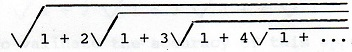# »  Solutions to puzzles in my VDARE.com monthly Diary

##July 2017

—————————

My July diary included the following brainteaser.

Does √(1+2√(1+3√(1+4√(1+5√(1+ …))))) have a finite numerical value? If so, what is it?

The expression in question looks neater if the square roots are written with overlines. Unfortunately that is beyond my HTML-coding capabilities, but here's an image:I described this in the diary as "an old chestnut." For sure it's at least 106 years old. It was posed by Srinivasa Ramanujan as Question 289 in Volume 3 of the Journal of the Indian Mathematical Society (1911). The background to that, although not the solution I give below, is in Chapter Three of Robert Kanigel's 1991 book about Ramanujan.

It's a cute problem, under the heading "deceptively simple." People not familiar with it get into all sorts of trouble. I've seen them resorting to advanced calculus.

Kanigel writes:

Three issues of the Journal came and went — six months — with no solution offered; in the end, Ramanujan supplied it himself.

—————————

• Solution

I shall give boldface Roman numbers and sub-numbers to all my key statements here.

The first statement is true for any positive real number a, by definition:

I.i    a = √(a²)

Rewriting that slightly:

I.ii    a = √(1 + (a² − 1))

Using a well-known bit of junior-high algebra, I can rewrite that as:

I.iii    a = √(1 + (a − 1)(a + 1))

Since that is true for any number a, it's just as true for a + 1. So, substituting a + 1 for a in I.iii:

II    a + 1 = √(1 + a(a + 2))

I.iii is also true for a + 2, of course. So, substituting a + 2 for a in I.iii:

III    a + 2 = √(1 + (a + 1)(a + 3))

This also works for a + 3 …

IV    a + 3 = √(1 + (a + 2)(a + 4))

… for a + 4 …

V    a + 4 = √(1 + (a + 3)(a + 5))

… and so on.

Now substitute the value of a + 1 given by II back into I.iii:

I.iv    a = √(1 + (a − 1)√(1 + a(a + 2)))

Substitute the value of a + 2 given by III back into that to get I.v; substitute the value of a + 3 given by IV into I.v to get I.vi, and … keep going.

You end up with an infinitely long expression which, if you set a = 3, is the one to be evaluated. The answer is therefore a = 3.

As I said, deceptively simple. The hardest math here is that bit of junior-high algebra.

No, that's not right. The hardest math here is seeing your way to a simple and elegant solution. That is genius-level math.